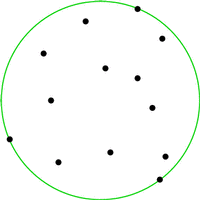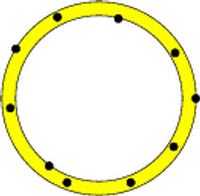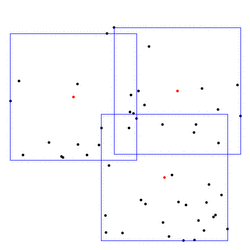CGAL 5.4.4 - Bounding Volumes
User ManualThis chapter describes algorithms which for a given point set compute the best circumscribing object from a specific class. If the class consists of all spheres in $$d$$-dimensional Euclidean space and best is defined as having smallest radius, then we obtain the smallest enclosing sphere.

# Introduction

Bounding volumes can be used to obtain simple approximations of complicated objects. For example, consider the problem of deciding whether two moving polygons currently intersect. An obvious solution is to discretize time and perform a full intersection test for any time step. If the polygons are far apart most of the time, this is unnecessary. Instead, simple bounding volumes (for examples, circles) are computed for both polygons at their initial positions. At subsequent time steps, an intersection test between the moving bounding circles replaces the actual intersection test; only if the circles do intersect, the expensive intersection test between the polygons is performed. In practice, bounding volume hierarchies are often used on top of simple bounding volumes to approximate complicated objects more accurately.

Bounding volumes are also frequently applied to extract geometric properties of objects. For example, the smallest enclosing annulus of a point set can be used to test whether a set of points is approximately cospherical. Here, the width of the annulus (or its area, or still another criterion that we use) is a good measure for this property.

# Bounding Spheres in dD

We provide the class Min_sphere_of_spheres_d<Traits> for arbitrary dimensions to compute the smallest enclosing spheres for points as well as for spheres. The dimension as well as the input type depend on the chosen traits class.

The following example is for 2D points

#include <CGAL/Simple_cartesian.h>
#include <CGAL/Min_sphere_of_spheres_d.h>
#include <CGAL/Min_sphere_of_points_d_traits_2.h>
#include <CGAL/Random.h>
#include <iostream>
typedef K::Point_2 Point;
int
main( int, char**)
{
const int n = 100;
Point P[n];
CGAL::Random r; // random number generator
for ( int i = 0; i < n; ++i){
P[ i] = Point(r.get_double(), r.get_double());
}
Min_circle mc( P, P+n);
Min_circle::Cartesian_const_iterator ccib = mc.center_cartesian_begin(), ccie = mc.center_cartesian_end();
std::cout << "center:";
for( ; ccib != ccie; ++ccib){
std::cout << " " << *ccib;
}
return 0;
}

The example for 2D circles as input looks rather similar.

// Computes the minsphere of some random spheres.
#include <CGAL/Cartesian.h>
#include <CGAL/Random.h>
#include <CGAL/Exact_rational.h>
#include <CGAL/Min_sphere_of_spheres_d.h>
#include <vector>
const int N = 1000; // number of spheres
const int LOW = 0, HIGH = 10000; // range of coordinates and radii
typedef CGAL::Exact_rational FT;
//typedef double FT;
typedef K::Point_2 Point;
typedef Traits::Sphere Sphere;
int main () {
std::vector<Sphere> S; // n spheres
CGAL::Random r; // random number generator
for (int i=0; i<N; ++i) {
const FT x = r.get_int(LOW,HIGH),
y = r.get_int(LOW,HIGH);
Point p(x,y); // random center...
}
Min_sphere ms(S.begin(),S.end()); // check in the spheres
CGAL_assertion(ms.is_valid());
}

## Bounding Spheres for the Homogeneous Kernel

In the previous section we saw that we used Min_sphere_of_spheres_d to compute the smallest circle for points. This package also provides the classes Min_circle_2 and Min_sphere_d, but they are slower, and they should only be used in case of homogeneous coordinates which are not supported by Min_sphere_of_spheres_d.

In the following example a smallest enclosing circle (Min_circle_2<Traits>) is constructed from points on a line and written to standard output. The example shows that it is advisable to switch on random shuffling in order to deal with a bad order of the input points.

#include <CGAL/Exact_integer.h>
#include <CGAL/Simple_homogeneous.h>
#include <CGAL/Min_circle_2.h>
#include <CGAL/Min_circle_2_traits_2.h>
#include <iostream>
// typedefs
typedef CGAL::Exact_integer RT;
typedef CGAL::Min_circle_2<Traits> Min_circle;
typedef K::Point_2 Point;
int
main( int, char**)
{
const int n = 100;
Point P[n];
for ( int i = 0; i < n; ++i){
P[i] = Point( (i%2 == 0 ? i : -i), 0, 1);
// (0,0), (-1,0), (2,0), (-3,0), ...
}
Min_circle mc1( P, P+n, false); // very slow
Min_circle mc2( P, P+n, true); // fast
std::cout << mc2;
return 0;
}

# Bounding Annulus in dD

We provide the class Min_annulus_d<Traits> for arbitrary dimensions to compute the smalles enclosing annulus for a set of points. In 2D the annulus consists of two concentric circles, in 3D of two concentric spheres.# Various Bounding Areas in 2D

Other classes for which we provide solutions are ellipses (Min_ellipse_2<Traits>), rectangles (min_rectangle_2()), parallelograms (min_parallelogram_2()) and strips (min_strip_2()) in the plane, with appropriate optimality criteria.

# Approximate Bounding Ellipsoid in dD

While this package provides an exact smallest 2D ellipse, it also provides the class Approximate_min_ellipsoid_d<Traits> to compute an approximate minimum-volume enclosing ellipsoid with user-specified approximation ratio.

# Rectangular P-Center

Bounding volumes also define geometric "center points" of objects. For example, if two objects are to be matched (approximately), one approach is to first apply the translation that maps the centers of their smallest enclosing spheres onto each other. Simpler centers are possible, of course (center of gravity, center of bounding box), but more advanced bounding volumes might give better results in some cases. It can also make sense to consider several center points instead of just one. For example, we provide algorithms to cover a planar point set with between two and four minimal boxes (rectangular_p_center_2()). Below is an example covering with three boxes; the center points are shown in red.